# Cost structure of a firm

This page describes some general aspects of the theory of the cost structure of a firm. For simplicity, we make the following assumptions:

• We consider a firm producing a single good.
• We ignore the question of whether the firm can store excess stock and thereby have some way of recovering costs if it overproduces; our analysis is related to production at a given stage in time.
• We consider only a two-level distinction between the short run (where some types of costs, called fixed costs, cannot be changed) and the long run (where all costs can be freely chosen). In practice, different cost decisions have different time lags and timeframes, but the simplistic short versus long run model will suffice for illustration.

We will consider the following constructs:

Function Acronym Units for input Units for output Definition
Production function of the firm -- Money (there are multiple inputs, one for each factor of production) Quantity The production function of the firm, which describes the firm's quantity produced as a function of the costs incurred by the factors of production.
Optimized production function of the firm -- Money Quantity This describes the firm's quantity produced as a function of total cost incurred assuming that the cost is allocated optimally between the various factors of production to maximize the quantity produced. We can distinguish here between the short-run optimized production function (this assumes that the fixed costs cannot be changed, and only allows for variation in the variable part of the costs) and the long-run optimized production function.
Total cost function (separate short-run and long-run versions) TC Quantity Money This is the inverse function to the optimized production function. Explicitly, it describes the cost of producing a given quantity as a function of quantity. We can distinguish between the short-run total cost function (the inverse to the short-run optimized production function) and the long-run total cost function (the inverse of the long-run optimized production function).
Fixed cost FC -- Money The total amount of cost that is fixed in the short run, for a given fixed cost scenario.
Variable cost function (short-run only) VC Quantity Money Given a fixed cost scenario (i.e., a particular choice of allocation of fixed costs that cannot be changed in the short run), the variable cost function is the function that describes the variable cost of producing a given quantity as a function of quantity. It is obtained from the short-run total cost function by subtracting the (constant) fixed cost from it.
Average cost function, aka average total cost function (separate short-run and long-run versions) AC, ATC Quantity Money per unit quantity Obtained by dividing the total cost function by the quantity. The short-run average cost function is obtained from the short-run total cost function, whereas the long-run average cost function is obtained from the long-run total cost function.
Average variable cost function (short-run only) AVC Quantity Money per unit quantity Obtained by dividing the variable cost function by the quantity.
Marginal cost function (separate short-run and long-run versions) MC Quantity Money per unit quantity Obtained by differentiating the total cost function (short-run for short-run, long-run for long-run). The short-run marginal cost function can also be obtained by differentiating the (short-run) variable cost function instead of the (short-run) total cost function, because these differ by a constant (the fixed cost value).

The entire analysis of the firm's cost structure is derived from the following two pieces of information:

• The production function of the firm.
• Information about what factors of production (or aspects of these factors) are part of the fixed cost scenario, and what factors of production are part of the variable cost scenario.

## The "capital as fixed cost, labor as variable cost" setting

A common setting is to choose two factors of production, capital (with expenditure on capital denoted$K$) and labor (with expenditure on labor denoted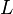$L$), and assume that the choice of$K$ describes the fixed cost scenario whereas$L$ is the variable cost. Explicitly,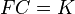$FC = K$ and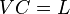$VC = L$. Note that this assumption is not appropriate for all settings: difficulties with hiring or firing people, and inflexibility in changing the number of hours worked by people, can lead to labor being a fixed cost in the short run, whereas easy ability to hire or rent capital resources can make capital a variable cost in the short run. Nonetheless, the assumption is valuable in many settings and can help illustrate general aspects of how production functions operate.

## The example of a Cobb-Douglas production function

### The production function and optimized production function

Suppose the quantity produced by the firm has the form:$Q = AK^\alpha L^\beta$

where$A$ is a positive constant, and$\alpha$ and$\beta$ denote the output elasticities with respect to the capital input$K$ and labor input$L$ respectively.

For a given cost$C$, the maximum quantity$Q$ can be produced if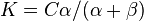$K = C \alpha/(\alpha + \beta)$ and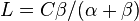$L = C \beta/(\alpha + \beta)$ (this is a simple calculus optimization problem). Thus, the optimized production function is: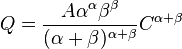$Q = \frac{A \alpha^\alpha \beta^\beta}{(\alpha + \beta)^{\alpha + \beta}} C^{\alpha + \beta}$

In particular, it is a power function (and hence, has constant elasticity). We can make three cases:

•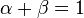$\alpha + \beta = 1$: The quantity produced scales linearly with the cost. We call this case constant returns to scale or a constant cost industry.
•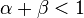$\alpha + \beta < 1$: The quantity produced scales sublinearly with the cost. We call this case decreasing returns to scale or an increasing cost industry.
•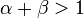$\alpha + \beta > 1$: The quantity produced scales superlinearly with the cost. We call this case increasing returns to scale or a decreasing cost industry.

### The total cost function

The total cost can be expressed in terms of the quantity by manipulating the above equation to solve for$C$. We obtain the following expression for total cost:$(\alpha + \beta)\left(\frac{Q}{A\alpha^\alpha \beta^\beta}\right)^{1/(\alpha + \beta)}$

Note in particular that the exponent on$Q$ is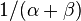$1/(\alpha + \beta)$, the reciprocal of the exponent for the optimized production function. This explains why the adjective used to describe the returns to scale and the adjective used to describe the cost function are opposites (decreasing returns to scale correspond to increasing cost industries, and increasing returns to scale correspond to decreasing cost industries).

### The average cost and marginal cost functions

The (long-run) average (total) cost function is obtained by dividing the total cost function by the quantity, so it is given as: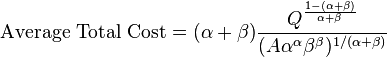$\mbox{Average Total Cost} = (\alpha + \beta)\frac{Q^{\frac{1 - (\alpha + \beta)}{\alpha + \beta}}}{(A \alpha^\alpha \beta^\beta)^{1/(\alpha + \beta)}}$

In particular:

•$\alpha + \beta = 1$:$Q$ disappears from the right side, so the average total cost is a constant function of quantity.
•$\alpha + \beta > 1$:$Q$ is raised to a negative exponent, indicating that the average total cost decreases with quantity (that is why the industry is called a decreasing cost industry).
•$\alpha + \beta < 1$:$Q$ is raised to a positive exponent, indicating that the average total cost increases with quantity.

The (long-run) marginal cost function is obtained by differentiating the total cost function with respect to quantity, so it is given as: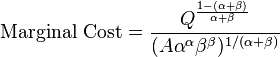$\mbox{Marginal Cost} = \frac{Q^{\frac{1 - (\alpha + \beta)}{\alpha + \beta}}}{(A \alpha^\alpha \beta^\beta)^{1/(\alpha + \beta)}}$

Note in particular that the marginal cost function in this case is proportional to the average total cost, with the proportionality constant being$1/(\alpha + \beta)$. This means that, except in the case$\alpha + \beta = 1$ (where they are identical and constant) the marginal cost curve and average total cost curve do not intersect. Cases:

•$\alpha + \beta = 1$:$Q$ disappears from the right side, so the marginal cost is a constant function of quantity and equals the average total cost
•$\alpha + \beta > 1$:$Q$ is raised to a negative exponent, indicating that the marginal cost decreases with quantity (that is why the industry is called a decreasing cost industry). Moreover, the marginal cost is always less than the average total cost (that's why the average total cost goes down with quantity).
•$\alpha + \beta < 1$:$Q$ is raised to a positive exponent, indicating that the average total cost increases with quantity. Moreover, the marginal cost is always greater than the average total cost (that's why the average total cost goes down with quantity).

### Short-run choice: short-run total cost function and short-run variable cost function, treating capital input as fixed and labor input as variable

We follow the assumption of treating capital input as fixed and labor input as variable in the short run. We have:$Q = AK^\alpha L^\beta$

Solving for$L$ in terms of$Q$, we obtain: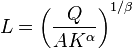$L = \left(\frac{Q}{AK^\alpha}\right)^{1/\beta}$

This is the short-run variable cost function. Explicitly:$\mbox{Variable cost} = \left(\frac{Q}{AK^\alpha}\right)^{1/\beta}$

The short-run total cost function is: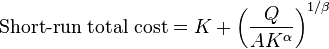$\mbox{Short-run total cost} = K + \left(\frac{Q}{AK^\alpha}\right)^{1/\beta}$

Consider the cases:

•$\beta < 1$: In this case, there are decreasing returns on labor alone, and therefore, the short-run total cost function is increasing at an increasing rate (this can be framed in terms of the average variable cost or the marginal cost function, which we turn to).
•$\beta = 1$: In this case, there are constant returns on labor alone, and therefore, the short-run total cost function is linear in quantity produced.
•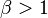$\beta > 1$: In this case,there are increasing returns on labor alone, and therefore ,the short-run total cost function is increasing at a decreasing rate.

### Short-run average variable cost and marginal cost function

The average variable cost function is given as: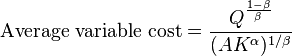$\mbox{Average variable cost} = \frac{Q^{\frac{1 - \beta}{\beta}}}{(AK^\alpha)^{1/\beta}}$

The short-run marginal cost function is given as: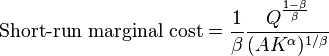$\mbox{Short-run marginal cost} = \frac{1}{\beta}\frac{Q^{\frac{1 - \beta}{\beta}}}{(AK^\alpha)^{1/\beta}}$

In particular, the short-run marginal cost function and average variable cost function are in constant proportion ($\beta$) and therefore, unless$\beta = 1$, they do not intersect. The above cases comparing$\beta$ with 1 can be better understood based on these expressions.

### Typical case

The typical case is where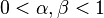$0 < \alpha,\beta < 1$ and$\alpha + \beta = 1$. Therfore:

• The long-run total cost curve is linear, and the long-run average cost curve and marginal cost curve are both horizontal (i.e., the corresponding functions are constant).
• The short-run total cost curve is concave up (convex), and the short-run average variable cost curve and marginal cost curve are both upward-sloping (i.e., the corresponding functions are increasing).